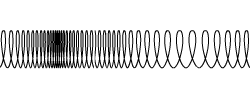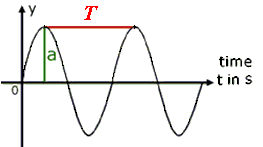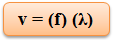Click to Chat

1800-1023-196

+91-120-4616500

CART 0

• 0

MY CART (5)

Use Coupon: CART20 and get 20% off on all online Study Material

ITEM
DETAILS
MRP
DISCOUNT
FINAL PRICE
Total Price: Rs.

There are no items in this cart.
Continue Shopping• Complete Physics Course - Class 11
• OFFERED PRICE: Rs. 2,968
• View Details

```Mechanical Wave Motion

Wave Motion Definition:-A waves motion can be defined as a disturbance that travels through a medium from one place to another. We consider the case of a slinky wave. When the slinky is stretched from end to end and is held at rest, it assumes an equilibrium position which is the position of rest. In order to induce a wave in slinky we first displace a particle of slinky from its position of rest. Wherever we move the coil whether upward or downward, forward or backward, it returns to its original position. But this movement creates a disturbance. If the slinky was moved in a back and forth direction then the disturbance observed in the slinky is called a slinky pulse. A pulse is a single disturbance that moves through a medium form one place to another. However, if the first coil of the slinky is continuously and periodically vibrated in a back-and-forth manner, it induces a repeating disturbance that continues for a longer duration. This disturbance is termed as a wave.

Frequency and Period of Wave:-The frequency of a wave refers to how often the particles of the medium vibrate when a wave passes through the medium. In mathematical terms, the frequency is the number of complete vibrational cycles of a medium per a given amount of time. The unit of frequency is the Hertz (abbreviated Hz) where 1 Hz is equivalent to 1 cycle/second. If a coil of slinky makes 2 vibrational cycles in one second, then the frequency is 2 Hz. If a coil of slinky makes 3 vibrational cycles in one second, then the frequency is 3 Hz.

The period of a wave is the time for a particle on a medium to make one complete vibrational cycle. Period, being a time, is measured in units of time such as seconds, hours, days or years. The period of orbit for the Earth around the Sun is approximately 365 days; it takes 365 days for the Earth to complete a cycle.

Types of  Wave Motion:-

Waves come in various shapes and forms. Though the basic characteristics of wave  motion are same and present in all waves but they can be distinguished on the basis of some distinguishing features.

Transverse Wave Mtion:-The wave in which particles of the medium move in a direction perpendicular to the direction of the wave is called a transverse wave. Now again if we consider the case of a slinky then if it is stretched in a horizontal direction and a movement is produced in the first coil by moving it up and down, energy is transported from left to right. Since the movement of particles is perpendicular to the direction of movemnt of wave so it is an example of traneverse wave.

Transverse wave motion is that wave motion in which particles of the medium execute SHM about their mean positions in a direction perpendicular to the direction of propagation of the wave. Such waves are called transverse waves. Examples of transverse waves are waves produced by plucked strings of veena, sitar or violin and electromagnetic waves. Transverse waves travel in the form of crests and troughs. The maximum displacement of the particle in the positive direction i.e. above its mean position is called crest and maximum displacement of the particle in the negative direction i.e below its mean position is called trough.

Thus if ABCDEFG is a transverse wave, the points B and F are crests while D is trough as shown in figure.For the propagation of transverse waves, the medium must possess force of cohesion and volume elasticity. Since gases and liquids do not have rigidity (cohesion), transverse waves cannot be produced in gases and liquids. Transverse waves can be produced in solids and surfaces of liquids only. Waves can also be categorized on the basis of their capability of transferring energy through a vacuum.

Longitudinal Wave Motion:-

The wave in which the particles move in a direction parallel to the direction of the movement of the wave is called a longitudinal wave. As discussed in the last case, in a slinky, once a disturbance is produced, the energy is transported from left to right. The particles of the medium move in a direction parallel to that of the pulse. Hence, such waves are longitudinal waves.

‘Longitudinal wave motion is that wave motion in which each particle of the medium executes simple harmonic motion about its mean position along the direction of propagation of the wave.’

Sound waves in fluids (liquids and gases) are examples of longitudinal wave. When a longitudinal wave travels through a medium, it produces compressions and rarefactions.In the case of a spiral spring, whose one end is tied to a hook of a wall and the other end is moved forward and backward, the coils of the spring vibrate about their original position along the length of the spring and longitudinal waves propagate through the spring as shown in figure.

The regions where the coils are closer are said to be in the state of compression, while the regions where the coils are farther are said to be in the state of rarefaction.When we strike a tuning fork on a rubber pad, the prongs of the tuning fork begin to vibrate to and fro about their mean positions. When the prong A moves outwards to A1, it compresses the layer of air in its neighbourhood. As the compressed layer moves forward it compresses the next layer and a wave of compression passes through air. But when the prong moves inwards to A2, the particles of the medium which moved to the right, now move backward to the left due to elasticity of air. This gives rise to rarefaction

Thus a longitudinal wave is characterised by the formation of compressions and rarefactions following each other.

Longitudinal waves can be produced in all types of material medium, solids, liquids and gases. The density and pressure of the medium in the region of compression are more than that in the region of rarefaction.

A transverse wave y = 0.05sin(20πx – 50πt) in meters, is propagating along +ve X-axis on a string. A velocity of 5 cm/s at t = 0 along the +ve X-axis from a point where x = 5 cm. After 5 s the difference in the phase of its position is equal to

(a) 150π                (b) 250π

(c) -245π               (d) -5π

Solution :-

At t = 0, x = 5 cm, initial phase of insect

?i = (20π) (5) – (50π) (0) = 100π

After 5s insect will move a distance s = vt = 25 cm

So, it will be at X = 30 cm.

Phase at this instant,

?f = (20π) (30) – (50π) (5) = 350π

Thus, ?? = ?f – ?i = 350π – 100π = 250π

From the above observation we conclude that, option (b) is correct.In a wavemotion (transverse or longitudinal), particles do not move forward. It is only the disturbance which is communicated from one point to other.

Velocity of a particle and velocity of wave are not same. Velocity of particle varies with time while that of wave is constant.

Sound belongs to the category of longitudinal weave motion while light belongs to the category of transeverse wavemotion.

Sound requires a material medium for its propagation, while light does not do so.

Electromagnetic wave Motion:-

Waves which are capable of transmitting energy through a vacuum and are produced by the vibration of charged particles are called electromagnetic waves. Light waves are an example of these waves. Electromagnetic waves are produced on Sun and travel to Earth through vacuum. These waves are responsible for the existence of life on Earth.

Mechanical Wave Motion:-

Those waves which cannot transmit their energy through a vacuum and require a medium for same are called mechanical waves. Various examples include sound waves, water waves, slinky waves etc.

Equation of Wave Motion:-

The wave motion equation can be expressed as

Speed = Wavelength • Frequency

It states the mathematical relationship between the speed (v) of a wave and its wavelength () and frequency (f). Using the symbols v, λ , and f, the equation can be rewritten asQuestion 1

Sound waves in a gas are always:

(a) transverse              (b) longitudinal

(c) stationary              (d) waves of electric and magnetic

Question 2

Light belongs to the category of:

(a) transverse wave motion          (b) longitudinal wave motion

(c) none of the above                   (d) (a) and (b)

Question 3

When sound travels from air to water, the quantity that remains unchanged is:

(a) speed            (b) frequency

(c) intensity         (d) wavelength

Question 4

If we throw a stone in a pond of standing water, the waves produced are:

(a) transverse             (b) longitudinal

(c) some other type    (d) (a) and (b)

Question 5

Two waves of same frequency and velocity should have:

(a) same wavelength

(b) different wavelengths

(c) sometimes same and sometimes different wavelengths

(d) none of the aboveQ.1
Q.2
Q.3
Q.4
Q.5

b

a

b

a

c

Related Resources:-

You might like to refer Center of Mass.

For getting an idea of the type of questions asked, refer the  Previous Year Question Papers.

To read more, Buy study material of Waves comprising study notes, revision notes, video lectures, previous year solved questions etc. Also browse for more study materials on Physics here.
```### Course Features

• 101 Video Lectures
• Revision Notes
• Previous Year Papers
• Mind Map
• Study Planner
• NCERT Solutions
• Discussion Forum
• Test paper with Video Solution